Question

# An analytical chemist is titrating 118.8 mL of a 0.2500 M solution of nitrous acid (HNO)...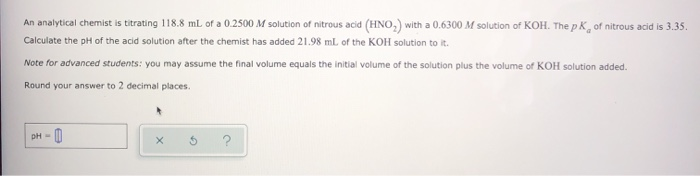An analytical chemist is titrating 118.8 mL of a 0.2500 M solution of nitrous acid (HNO) with a 0.6300 M solution of KOH. The pk of nitrous acid is 3.35. Calculate the pH of the acid solution after the chemist has added 21.98 ml of the KOH solution to it. Note for advanced students: you may assume the final volume equals the initial volume of the solution plus the volume of KOH solution added. Round your answer to 2 decimal places. pH-

pKa of acid = 3.35

HNO2 + KOH ---------------------> KNO2 + H2O

118.8x0.25=29.7 21.98x0.63=13.85 0 0 initial mmoles

15.85 0 13.85 ----------equilibrium

thus the solution is a buffer whose pH is given by Hendersen equation as

pH = pKa + log [conjugate base]/[acid]

= 3.35 + log [13.85/15.85]

= 3.29

#### Earn Coins

Coins can be redeemed for fabulous gifts.

Similar Homework Help Questions
• ### An analytical chemist is titrating 201.8 mL of a 0.5300 M solution of nitrous acid (HNO, with a 1.100 M solution of KOH...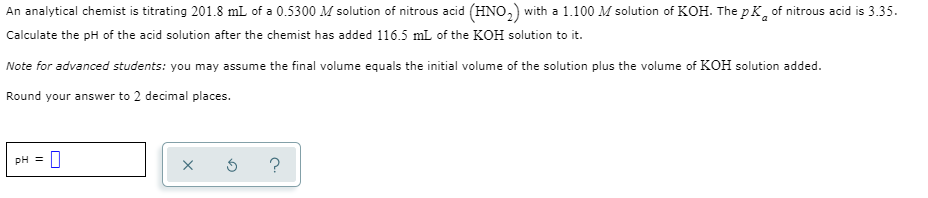An analytical chemist is titrating 201.8 mL of a 0.5300 M solution of nitrous acid (HNO, with a 1.100 M solution of KOH. The pk of nitrous acid is 3.35. Calculate the pH of the acid solution after the chemist has added 116.5 mL of the KOH solution to it. Note for advanced students: you may assume the final volume equals the initial volume of the solution plus the volume of KOH solution added. Round your answer to 2 decimal...

• ### An analytical chemist is titrating 228.6 mL of a 0.3500 M solution of nitrous acid (HNO.)...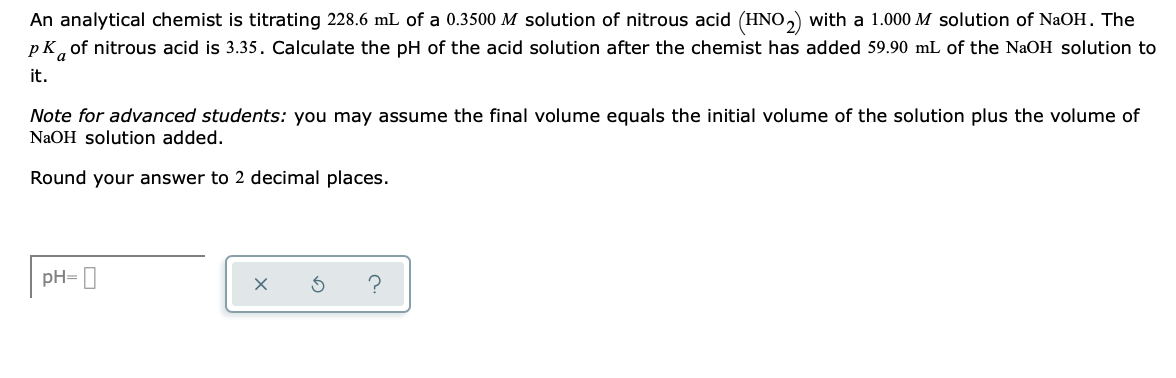An analytical chemist is titrating 228.6 mL of a 0.3500 M solution of nitrous acid (HNO.) with a 1.000 M solution of NaOH. The pk, of nitrous acid is 3.35. Calculate the pH of the acid solution after the chemist has added 59.90 mL of the NaOH solution to it. Note for advanced students: you may assume the final volume equals the initial volume of the solution plus the volume of NaOH solution added. Round your answer to 2 decimal...

• ### An analytical chemist is titrating 91.8 mL of a 0.4200 M solution of nitrous acid (HNO2)...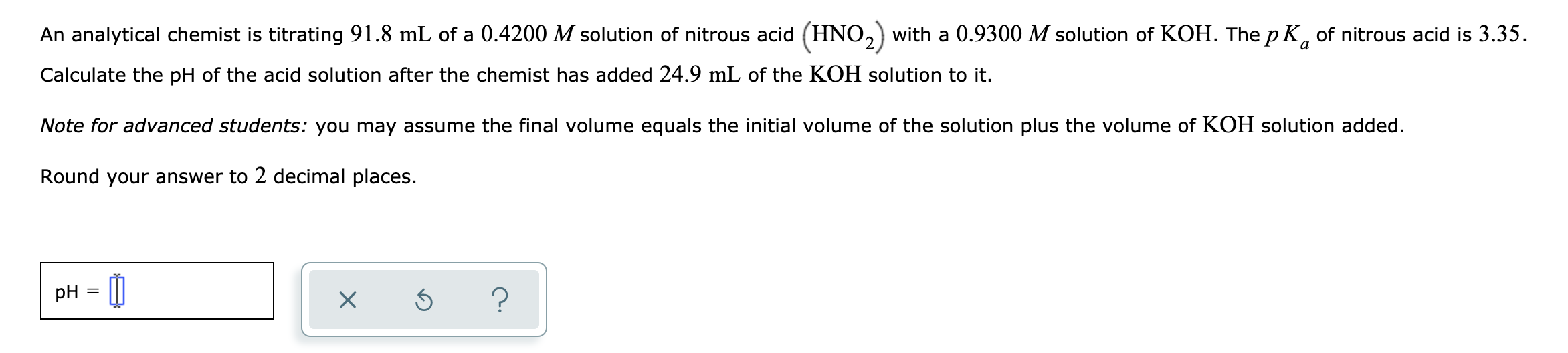An analytical chemist is titrating 91.8 mL of a 0.4200 M solution of nitrous acid (HNO2) with a 0.9300 M solution of KOH. The pK, of nitrous acid is 3.35. Calculate the pH of the acid solution after the chemist has added 24.9 mL of the KOH solution to it. Note for advanced students: you may assume the final volume equals the initial volume of the solution plus the volume of KOH solution added. Round your answer to 2 decimal...

• ### An analytical chemist is titrating 123.5 mL of a 0.9200 M solution of nitrous acid (HNO...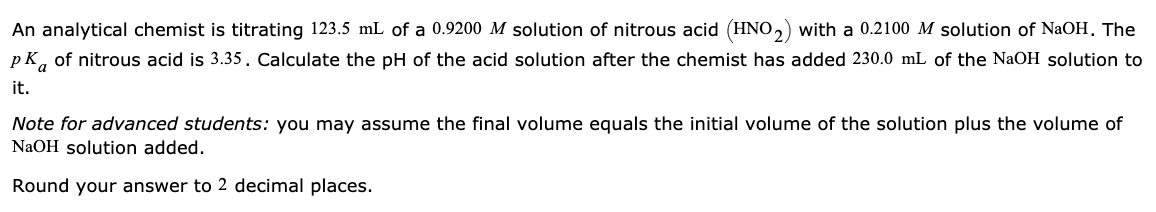An analytical chemist is titrating 123.5 mL of a 0.9200 M solution of nitrous acid (HNO 2) with a 0.2100 M solution of NaOH. The p K, of nitrous acid is 3.35. Calculate the pH of the acid solution after the chemist has added 230.0 mL of the NaOH solution to it. Note for advanced students: you may assume the final volume equals the initial volume of the solution plus the volume of NaOH solution added. Round your answer to...

• ### An analytical chemist is titrating 241.8mL of a 1.200M solution of nitrous acid HNO2 with a 0.5200M solution of NaOH. Th...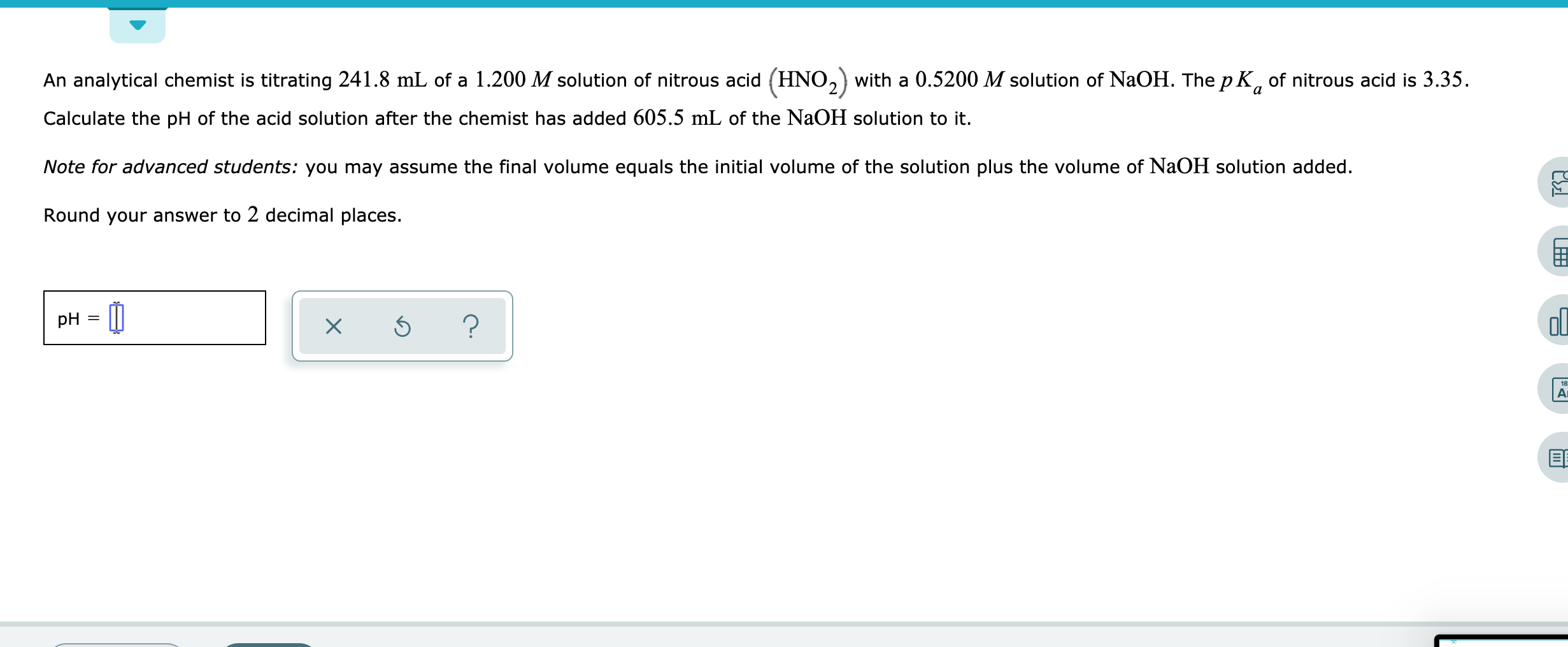An analytical chemist is titrating 241.8mL of a 1.200M solution of nitrous acid HNO2 with a 0.5200M solution of NaOH. The pKa of nitrous acid is 3.35. Calculate the pH of the acid solution after the chemist has added 605.5mL of the NaOH solution to it. Note for advanced students: you may assume the final volume equals the initial volume of the solution plus the volume of NaOH solution added. Round your answer to 2 decimal places. An analytical chemist...

• ### An analytical chemist is titrating 104.5 mL of a 1.000 M solution of formic aci with...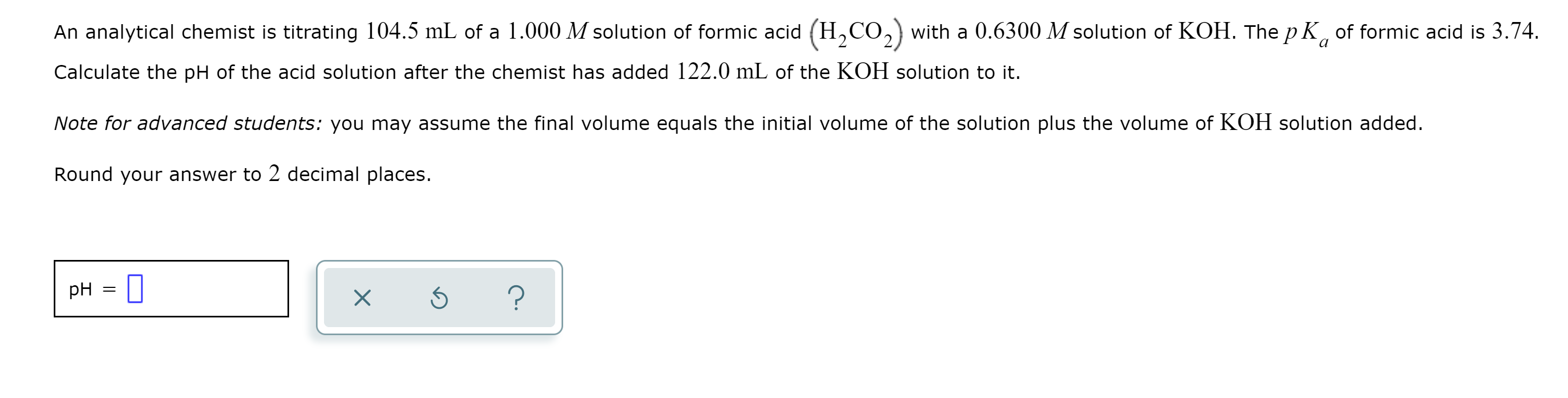An analytical chemist is titrating 104.5 mL of a 1.000 M solution of formic aci with a 0.6300 M solution of KOH. The pK of formic acid is 3.74. Calculate the pH of the acid solution after the chemist has added 122.0 mL of the KOH solution to it. Note for advanced students: you may assume the final volume equals the initial volume of the solution plus the volume of KOH solution added. Round your answer to 2 decimal places....

• ### An analytical chemist is titrating 72.8 ml. of a 0.6300 M solution of acetic acid (HCH,CO,) with a 0.6600 M solutio...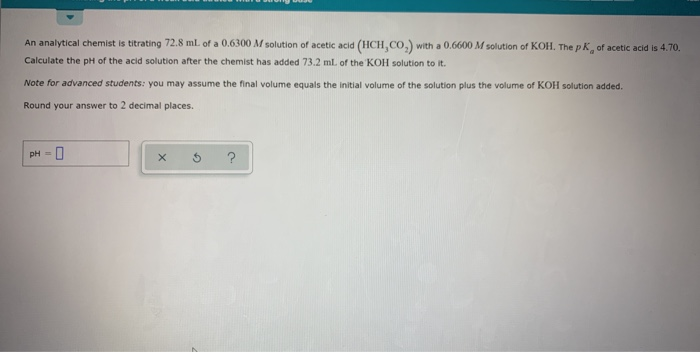An analytical chemist is titrating 72.8 ml. of a 0.6300 M solution of acetic acid (HCH,CO,) with a 0.6600 M solution of KOH. The pk, of acetic acid is 4.70. Calculate the pH of the acid solution after the chemist has added 73.2 ml of the KOH solution to it Note for advanced students: you may assume the final volume equals the initial volume of the solution plus the volume of KOH solution added. Round your answer to 2 decimal...

• ### - Calculating the pH of a weak acid titrated with a strong base An analytical chemist is titrating 123.0 ml. of a 0...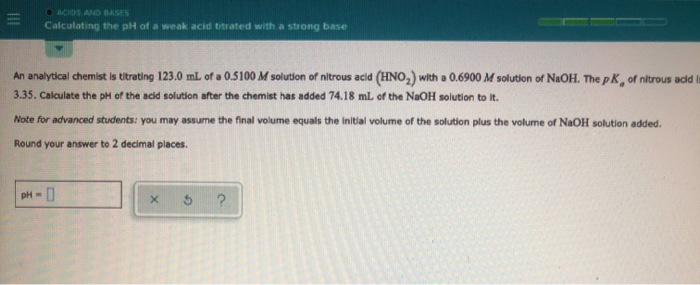- Calculating the pH of a weak acid titrated with a strong base An analytical chemist is titrating 123.0 ml. of a 0.5100 M solution of nitrous acid (HNO,) with a 0.6900 M solution of NaOH. The pk of nitrous add 3.35. Calculate the pH of the acid solution after the chemist has added 74.18 ml of the NaOH solution to it. Note for advanced students you may assume the final volume equals the initial volume of the solution plus...

• ### calculating the ph of a weak acid titrated with a strong base An analytical chemist is...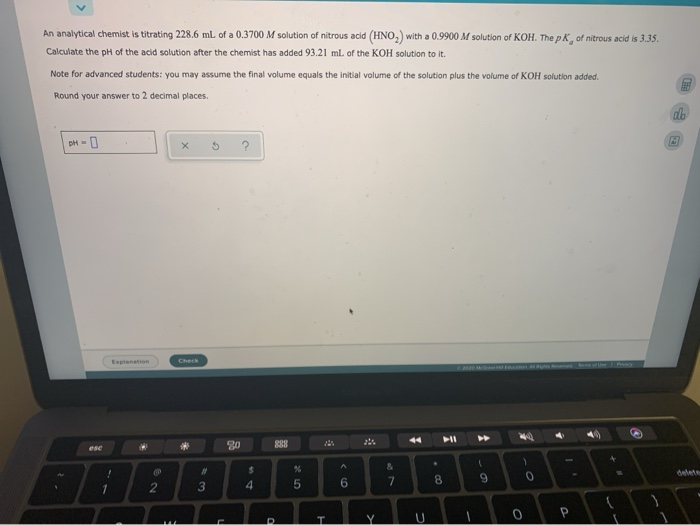calculating the ph of a weak acid titrated with a strong base An analytical chemist is titrating 228.6 ml. of a 0.3700 M solution of nitrous acid (HNO,) with a 0.9900 M solution of KOH. The pk of nitrous acid is 3.35. Calculate the pH of the acid solution after the chemist has added 93.21 ml of the KOH solution to it. Note for advanced students: you may assume the final volume equals the initial volume of the solution plus...

• ### Calculating the ph of a weak acid bitrated with a strong base An analytical Chemist is...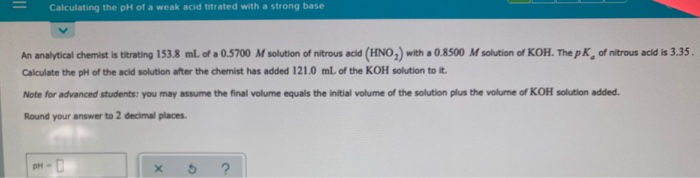Calculating the ph of a weak acid bitrated with a strong base An analytical Chemist is titrating 153.8 ml. of a 0.5700 M solution of nitrous acid (HNO,) with a 0.8500 M solution of KOH. The pk of nitrous acid is 3.35. Calculate the pH of the acid solution after the chemist has added 121.0 ml. of the KOH solution to it. Note for advanced students: you may assume the final volume equals the initial volume of the solution plus...